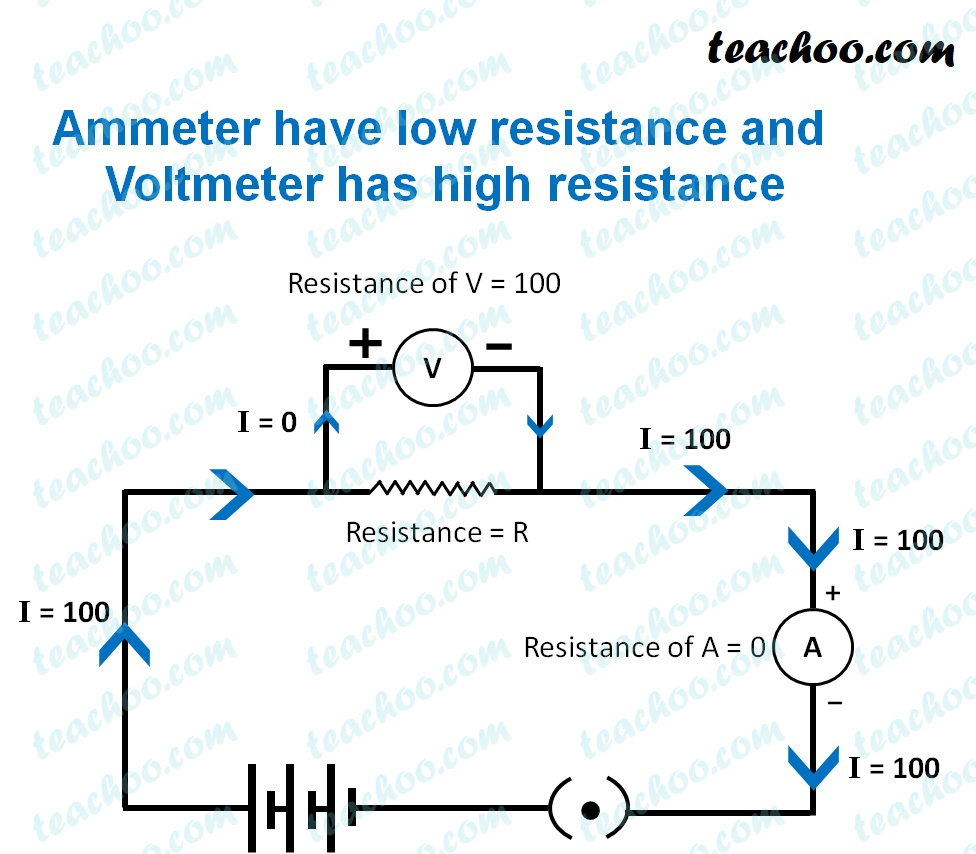Teachoo Questions

Class 10
Chapter 12 Class 10 - ElectricityA voltemeter is a device used to measure the amount of potential difference across a resistor or a combination of resitors.

• The resistance of an ideal voltmeter should be infinite.
• This is because a voltmeter is connected in parallel i n a circuit across resistor/resistors.

If the r esistance of the voltmeter won't be high , it would draw some current and the other resistors connected in parallel with it will not get enough current through them.

Hence, in order to avoid the change of current flowing through the resistor/resistors.

The resistance of a voltmeter should be infinitely high , so that it does not draw any current.

Learn in your speed, with individual attention - Teachoo Maths 1-on-1 Class